# 3.5: Naming Cycloalkanes

$$\newcommand{\vecs}{\overset { \rightharpoonup} {\mathbf{#1}} }$$ $$\newcommand{\vecd}{\overset{-\!-\!\rightharpoonup}{\vphantom{a}\smash {#1}}}$$$$\newcommand{\id}{\mathrm{id}}$$ $$\newcommand{\Span}{\mathrm{span}}$$ $$\newcommand{\kernel}{\mathrm{null}\,}$$ $$\newcommand{\range}{\mathrm{range}\,}$$ $$\newcommand{\RealPart}{\mathrm{Re}}$$ $$\newcommand{\ImaginaryPart}{\mathrm{Im}}$$ $$\newcommand{\Argument}{\mathrm{Arg}}$$ $$\newcommand{\norm}{\| #1 \|}$$ $$\newcommand{\inner}{\langle #1, #2 \rangle}$$ $$\newcommand{\Span}{\mathrm{span}}$$ $$\newcommand{\id}{\mathrm{id}}$$ $$\newcommand{\Span}{\mathrm{span}}$$ $$\newcommand{\kernel}{\mathrm{null}\,}$$ $$\newcommand{\range}{\mathrm{range}\,}$$ $$\newcommand{\RealPart}{\mathrm{Re}}$$ $$\newcommand{\ImaginaryPart}{\mathrm{Im}}$$ $$\newcommand{\Argument}{\mathrm{Arg}}$$ $$\newcommand{\norm}{\| #1 \|}$$ $$\newcommand{\inner}{\langle #1, #2 \rangle}$$ $$\newcommand{\Span}{\mathrm{span}}$$

Objectives

After completing this section, you should be able to

1. name a substituted or unsubstituted cycloalkane, given its Kekulé structure, shorthand structure or condensed structure.
2. draw the Kekulé, shorthand or condensed structure for a substituted or unsubstituted cycloalkane, given its IUPAC name.

Key Terms

Make certain that you can define, and use in context, the key terms below.

• cycloalkane

Study Notes

Provided that you have mastered the IUPAC system for naming alkanes, you should find that the nomenclature of cycloalkanes does not present any particular difficulties.

Many organic compounds found in nature contain rings of carbon atoms. These compounds are known as cycloalkanes. Cycloalkanes only contain carbon-hydrogen bonds and carbon-carbon single bonds. The simplest examples of this class consist of a single, un-substituted carbon ring, and these form a homologous series similar to the unbranched alkanes.

Like alkanes, cycloalkane molecules are often drawn as skeletal structures in which each intersection between two lines is assumed to have a carbon atom with its corresponding number of hydrogens. Cyclohexane, one of the most common cycloalkanes is shown below as an example.Cyclic hydrocarbons have the prefix "cyclo-". The IUPAC names, molecular formulas, and skeleton structures of the cycloalkanes with 3 to 10 carbons are given in Table 4.1.1. Note that the general formula for a cycloalkane composed of n carbons is CnH2n, and not CnH2n+2 as for alkanes. Although a cycloalkane has two fewer hydrogens than the equivalent alkane, each carbon is bonded to four other atoms so are still considered to be saturated with hydrogen.

Table $$\PageIndex{1}$$: Examples of Simple Cycloalkanes
Cycloalkane Molecular Formula Skeleton Structure
Cyclopropane C3H6Cyclobutane C4H8Cyclopentane C5H10Cyclohexane C6H12Cycloheptane C7H14Cyclooctane C8H16Cyclononane C9H18Cyclodecane C10H20## IUPAC Rules for Nomenclature

The naming of substituted cycloalkanes follows the same basic steps used in naming alkanes.

1. Determine the parent chain.
2. Number the substituents of the ring so that the sum of the numbers is the lowest possible.
3. Name the substituents and place them in alphabetical order.

More specific rules for naming substituted cycloalkanes with examples are given below.

1. Determine the cycloalkane to use as the parent. If there is an alkyl straight chain that has a greater number of carbons than the cycloalkane, then the alkyl chain must be used as the primary parent chain. Cycloalkanes substituents have an ending "-yl". If there are two cycloalkanes in the molecule, use the cycloalkane with the higher number of carbons as the parent.

Example $$\PageIndex{1}$$The longest straight chain contains 10 carbons, compared with cyclopropane, which only contains 3 carbons. The parent chain in this molecule is decane and cyclopropane is a substituent, . The name of this molecule is 3-cyclopropyl-6-methyldecane.

Example $$\PageIndex{2}$$

Name the cycloalkane structure.Solution

There are two different cycloalkanes in this molecule.  Because it contains more carbons, the cyclopentane ring will be named as the parent chain.  The smaller ring, cyclobutane, is named as a substituent on the parent chain.  The name of this molecule is cyclobutylcyclopentane.

2) When there is only one substituent on the ring, the ring carbon attached to the substituent is automatically carbon #1. Indicating the number of the carbon with the substituent in the name is optional.

Example $$\PageIndex{3}$$1-chlorocyclobutane or cholorocyclobutane 1-propylcyclohexane or propylcyclohexane

3) If there are multiple substituents on the ring, number the carbons of the cycloalkane so that the carbons with substituents have the lowest possible number. A carbon with multiple substituents should have a lower number than a carbon with only one substituent or functional group. One way to make sure that the lowest number possible is assigned is to number the carbons so that when the numbers corresponding to the substituents are added, their sum is the lowest possible.

4) When naming the cycloalkane, the substituents must be placed in alphabetical order. Remember the prefixes di-, tri-, etc. , are not used for alphabetization.

Example $$\PageIndex{4}$$In this example, the ethyl or the methyl subsistent could be attached to carbon one.  The ethyl group attachment is assigned carbon 1 because ethyl comes before methyl alphabetically.  After assigning carbon 1 the cyclohexane ring can be numbered going clockwise or counterclockwise.  When looking at the numbers produced going clockwise produces lower substituents numbers (4) than when numbered counterclockwise (6).

Example $$\PageIndex{5}$$

Name the following structure using IUPAC rules.Solution

Remember when dealing with cycloalkanes with more than two substituents, finding the lowest possible substituent numbering takes prescient.  Consider a numbering system with each substituent attachment point as being carbon one.  Compare them and whichever produces the lowest numbering will be correct.Because there are three substituents there are three possible numbering systems.  The one shown to the left allows for a distinctly lower numbering system.  Note, that alphabetical prioritizing was not used to make this assement.  The correct name for the molecule is 4-Bromo-1,2-dimethylcyclohesane.

Example $$\PageIndex{6}$$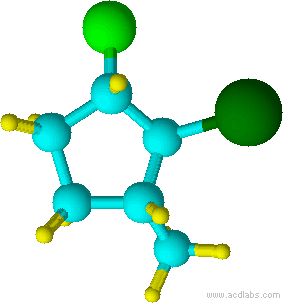2-bromo-1-chloro-3-methylcyclopentane

Notice that "b" of bromo alphabetically precedes the "m" of methyl.  Also, notice that the chlorine attachment point is assigned carbon 1 because it comes first alphabetically and the overall sum of numbers would be the same if the methyl attachment carbon was assigned as 1 and the chlorine attachment as 3.

Example $$\PageIndex{7}$$(2-bromo-1,1-dimethylcyclohexane)

Although "di" alphabetically precedes "f", "di" is not used in determining the alphabetical order.

Example $$\PageIndex{8}$$(2-fluoro-1,1,-dimethylcyclohexane NOT 1,1-dimethyl-2-fluorocyclohexane)

also

2-fluoro-1,1,-dimethylcyclohexane (2+1+1 = 4) NOT 1-fluoro-2,2-dimethylcyclohexane (2+2+1 = 5)

Although "di" alphabetically precedes "f", "di" is not used in determining the alphabetical order of the substitutents.  Notice that the attachment point of the two methyl groups is assigned carbon 1 despite the fact that fluorine comes first alphabetically. This is because this assignment allows for a lower overall numbering of substituents, so assigning alphabetical priority is not necessary.

Hydrocarbons having more than one ring are common, and are referred to as bicyclic (two rings), tricyclic (three rings) and in general, polycyclic compounds. The molecular formulas of such compounds have H/C ratios that decrease with the number of rings. In general, for a hydrocarbon composed of n carbon atoms associated with m rings the formula is: CnH(2n + 2 - 2m). The structural relationship of rings in a polycyclic compound can vary. They may be separate and independent, or they may share one or two common atoms. Some examples of these possible arrangements are shown in the following table.

Table $$\PageIndex{2}$$: Examples of Isomeric
Bicycloalkanes
Isolated Rings Spiro Rings Fused Rings Bridged Rings
No common atoms One common atom One common bond Two common atomsPolycyclic compounds, like cholesterol shown below, are biologically important and typically have common names accepted by IUPAC. However, the common names do not generally follow the basic IUPAC nomenclature rules, and will not be covered here.Cholesterol (polycyclic)

## Problems

Name the following structures. (Note: The structures are complex for practice purposes and may not be found in nature.)

1)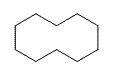2)3)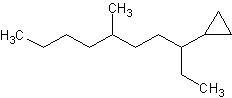4)5)6)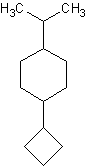Draw the following structures.

7) 1,1-dibromo-3-butyl-5-fluoro-7-methylcyclooctane

8) 1,1-dibromo-2,3-dichloro-4-propylcyclobutane

9) 1-ethyl-2-methyl-1,3-dipropylcyclopentane

Name the following structures.

Blue=Carbon Yellow=Hydrogen Green=Chlorine

10)11)12)13)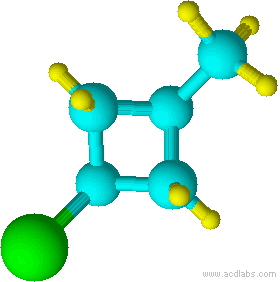1) cyclodecane 2) chlorocyclopentane or 1-chlorocyclopentane

3) 3-cyclopropyl-6-methyldecane 4) cyclopentylcyclodecane or 1-cyclopentylcyclodecane 5) 1,3-dibromo-1-chloro-2-fluorocycloheptane

6) 1-cyclobutyl-4-isopropylcyclohexane

7)8)9)10) cyclohexane 11) chlorocyclohexane 12) cyclopentylcyclohexane 13) 1-chloro-3-methylcyclobutane

## Contributors

This page titled 3.5: Naming Cycloalkanes is shared under a not declared license and was authored, remixed, and/or curated by Layne Morsch.